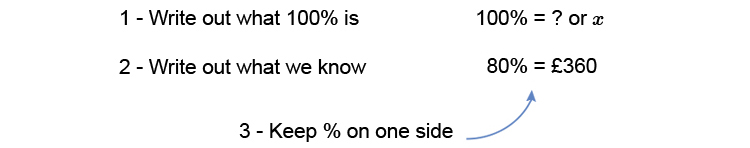# Finding the original number

Example 1

A car is sold for £360 after a discount of 20%. Find the original price.NOTE:

£360 after a discount of 20% is the same as saying £360 is 80% of the original price.Multiply both sides by 360 to get x on its own (remember – what ever you do to one side you do to the other).

(100times360)/80=(xtimescancel360)/cancel360

(100times360)/80=x

x=(100timescancel360\ \ \ 4.5)/cancel80

x=100times4.5=450

Answer: The original price is £450